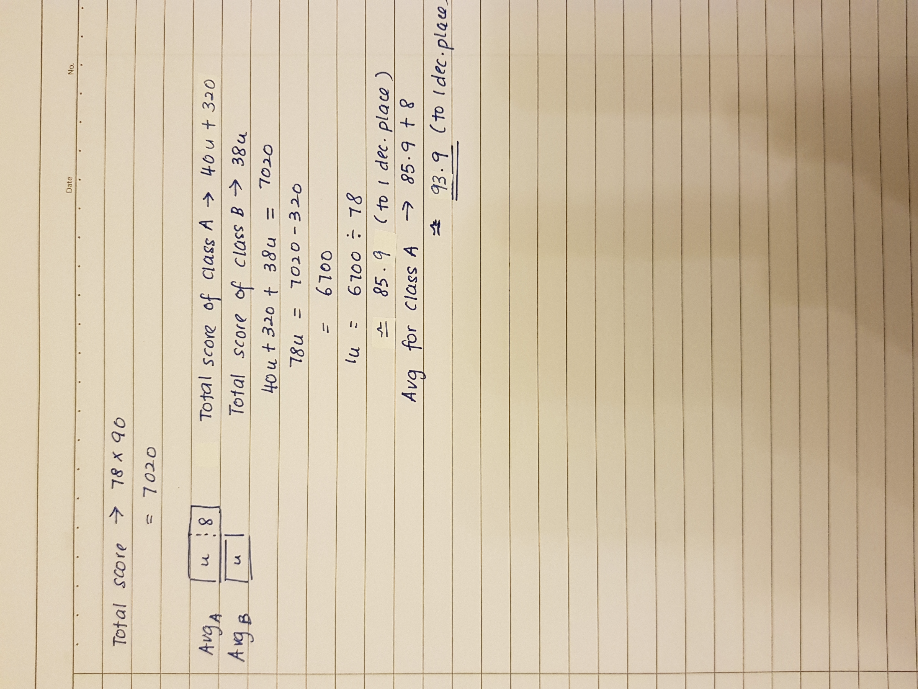# Question0 Replies 0 Likes

40 + 38 = 78
78 x 90 = 7020 (total score for both classes)

Method 1 :
38 x 8 = 304
7020 + 304 = 7324
7324/78 = 93.9 (correct to 1 dp)

Method 2 :
Assume the average score of Primary 6A as 1u
40 x 1u + 38 x (1u – 8) = 78u – 304 ——- 7324
1u ——- (7324 + 304)/78 = 93.9 (correct to 1 dp)

Ans : 93.9 (correct to 1 dp).

0 Replies 0 Likes